Home | | Business Maths 12th Std | Rank of a Matrix: Solved Example Problems

# Rank of a Matrix: Solved Example Problems

Business Maths and Statistics : Applications of Matrices and Determinants: Rank of a Matrix: Solved Example Problems with Answers, Solution and Explanation

Example 1.1

Find the rank of the matrixSolution:

Let A=Order of A is 2 û 2 ãÇ          ü(A)ãÊ 2

Consider the second order minor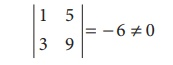There is a minor of order 2, which is not zero. ãÇü (A= 2

Example 1.2

Find the rank of the matrix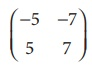Solution:

Let A=Order of A is 2 û 2 ãÇü(A)ãÊ 2

Consider the second order minorSince the second order minor vanishes, ü(Aã  2

Consider a first order minor |ã5| ã  0

There is a minor of order 1, which is not zero

ãÇ ü (A) = 1

Example 1.3

Find the rank of the matrixSolution:

Let A=Order Of A is 3x3

ãÇ ü (A) ãÊ 3

Consider the third order minor= 6 ã  0

There is a minor of order 3, which is not zero

ãÇü (A) = 3.

Example 1.4

Find the rank of the matrixSolution:

Let A=Order Of A is 3x3

ãÇ ü (A) ãÊ 3

Consider the third order minorSince the third order minor vanishes, therefore ü(Aã  3

Consider a second order minorThere is a minor of order 2, which is not zero.

ãÇ ü(A) = 2.

Example 1.5

Find the rank of the matrixSolution:

Let AOrder of A is 3 û 4

ãÇ ü(A)ãÊ 3.

Consider the third order minorsSince all third order minors vanishes, ü(Aã  3.

Now, let us consider the second order minors,

Consider one of the second order minorsThere is a minor of order 2 which is not zero.

ãÇü (A) = 2.

## Echelon form and finding the rank of the matrix (upto the order of 3û4) : Solved Example Problems

Example 1.6

Find the rank of the matrix A=Solution :

The order of A is 3 û 3.

ãÇ ü(A) ãÊ 3.

Let us transform the matrix A to an echelon form by using elementary transformations.The number of non zero rows is 2

ãÇRank of A is 2.

ü (A) = 2.

Note

A row having atleast one non -zero element is called as non-zero row.

Example 1.7

Find the rank of the matrix A=Solution:

The order of A is 3 û 4.

ãÇ ü (A)ãÊ3.

Let us transform the matrix A to an echelon formThe number of non zero rows is 3. ãÇ ü(A) =3.

Example 1.8

Find the rank of the matrix A=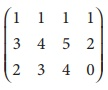Solution:

The order of A is 3 û 4.

ãÇ ü(A) ãÊ 3.

Let us transform the matrix A to an echelon form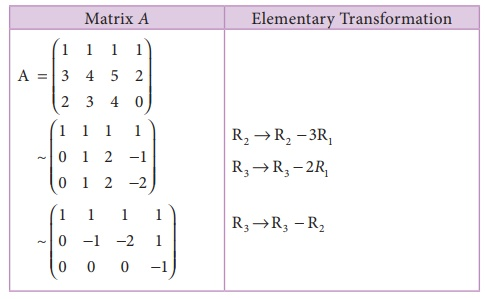The number of non zero rows is 3.

ãÇ ü (A) =3.

## Testing the consistency of non homogeneous linear equations (two and three variables) by rank method : Solved Example Problems

Example 1.9

Show that the equations x + y = 5, 2x + y = 8 are consistent and solve them.

Solution:

The matrix equation corresponding to the given system is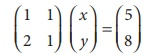AX=BNumber of non-zero rows is 2.

ü (A )= ü ([ A, B]) = 2 = Number of unknowns.

The given system is consistent and has unique solution.

Now, the given system is transformed into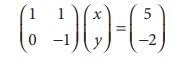+ y = 5

= 2

ãÇ (1) ã x + 2 = 5

x = 3

Solution is x = 3, y = 2

Example 1.10

Show that the equations 2x + y = 5, 4x + 2 y = 10 are consistent and solve them.

Solution:

The matrix equation corresponding to the system isü ( A ) = ü ([ A, B]) = 1 < number of unknowns

ãÇ The given system is consistent and has infinitely many solutions.

Now, the given system is transformed into the matrix equation.Let us take = k, k ãR

ã 2x + k = 5

x = 1/2 ( 5 ã k)

1/2 ( 5 ã k) , y = k for all k ãR

Thus by giving different values for k, we get different solution. Hence the system has infinite number of solutions.

Example 1.11

Show that the equations 3x ã 2 y = 6, 6x ã 4 y = 10 are inconsistent.

Solution:

The matrix equation corresponding to the given system is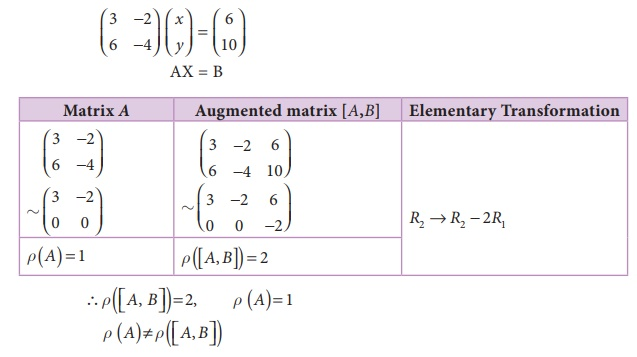ãÇThe given system is inconsistent and has no solution.

Example 1.12

Show that the equations 2x + y + z = 5, x + y + z = 4, x ã y + 2z = 1 are consistent and hence solve them.

Solution:

The matrix equation corresponding to the given system is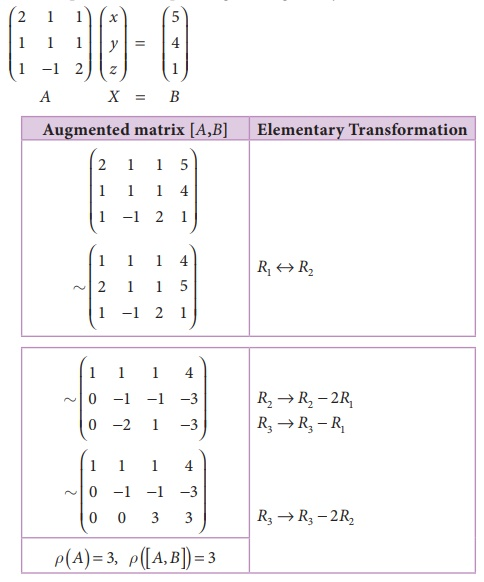Obviously the last equivalent matrix is in the echelon form. It has three non-zero rows.

ü( A ) = ü( [A, B] )= 3 = Number of unknowns .

The given system is consistent and has unique solution.

To find the solution, let us rewrite the above echelon form into the matrix form.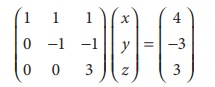x + y + z = 4 (1)

y + z = 3 (2)

3z = 3 (3)

(3)ã z = 1

(2)ã y = 3 ã z = 2

(1) ã x = 4 ã y ã z

x=1

ãÇ x = 1, y = 2, z = 1

Example 1.13

Show that the equations x + y + z = 6, x + 2 y + 3z = 14, x + 4 y + 7z = 30 are consistent and solve them.

Solution:

The matrix equation corresponding to the given system is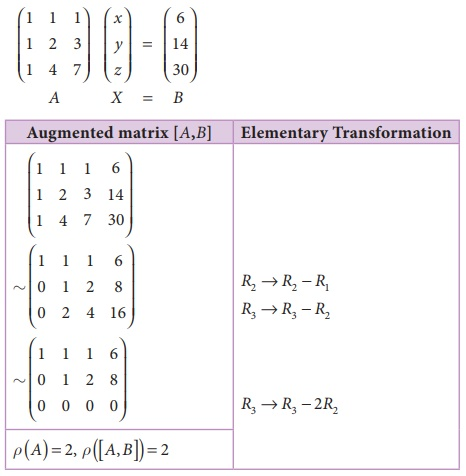Obviously the last equivalent matrix is in the echelon form. It has two non-zero rows.

ãÇ ü ( [A, B] ) = 2, ü ( A) = 2

ü ( A ) = ü ( [A, B] ) = 2 < Number of unknowns.

The given system is consistent and has infinitely many solutions.

The given system is equivalent to the matrix equation,x + y + z = 6  (1)

y + 2z = 8 (2)

(2)ã y = 8 ã 2z,

(1)ã x = 6 ã y ã z = 6 ã (8 ã 2 z) ã z = z ã 2

Let us take z = kk ãR , we get x = k ã 2, y = 8 ã 2k , Thus by giving different values for k we get different solutions. Hence the given system has infinitely many solutions.

Example 1.14

Show that the equations x ã 4 y + 7z = 14, 3x + 8 y ã 2z = 13, 7x ã 8 y + 26z = 5 are inconsistent.

Solution:

The matrix equation corresponding to the given system isThe last equivalent matrix is in the echelon form. [A, B] has 3 non-zero rows and [A] has 2 non-zero rows.The system is inconsistent and has no solution.

Example 1.15

Find k, if the equations x + 2 y ã 3z = ã2, 3x ã y ã 2z = 1, 2x + 3y ã 5z = k are consistent.

Solution:

The matrix equation corresponding to the given system isFor the equations to be consistent, ü ( [AB] ) = ü ( A)= 2

ãÇ21 + 7k = 0

7k = ã21 .

= ã3

Example 1.16

Find k, if the equations x + y + z = 7, x + 2 y + 3z = 18, y + kz = 6 are inconsistent.

Solution:

The matrix equation corresponding to the given system isFor the equations to be inconsistent

ü ( [A, B] ) ã  ü ( A)

It is possible if k ã 2 = 0 .

K=2

Example 1.17

Investigate for what values of ãaã and ãbã the following system of equations

x + y + z = 6, x + 2 y + 3z = 10, x + 2 y + az = b have

(i) no solution  (ii) a unique solution (iii) an infinite number of solutions.

Solution:

The matrix equation corresponding to the given system isCase (i) For no solution:

The system possesses no solution only when ü ( A )ã  ü ([ AB]) which is possible only when a ã 3 = 0 and b ã 10 ã  0

Hence for a = 3, b ã  10 , the system possesses no solution.

Case (ii) For a unique solution:

The system possesses a unique solution only when ü ( A ) = ü ([ AB]) = number of unknowns.

i.e when ü ( A ) = ü ([ AB]) = 3

Which is possible only when a ã 3 ã  0 and b may be any real number as we can observe .

Hence for a ã  3 and b ãR , the system possesses a unique solution.

Case (iii) For an infinite number of solutions:

The system possesses an infinite number of solutions only when

ü ( A )= ü ([ A, B]) < number of unknowns

i,e when ü ( A)= ü ([ AB])= 2 < 3 ( number of unknowns) which is possible only when a ã 3 = 0, b ã 10 = 0

Hence for a = 3, b =10, the system possesses infinite number of solutions.

Example 1.18

The total number of units produced (P) is a linear function of amount of over times in labour (in hours) (l), amount of additional machine time (m) and fixed finishing time (a)

i.e, P = a + bl + cm

From the data given below, find the values of constants a, b and cEstimate the production when overtime in labour is 50 hrs and additional machine time is 15 hrs.

Solution:

We have, P = a + bl + cm

Putting above values we have

6,950 = a + 40b + 10c

6,725 = a + 35b + 9c

7,100 = a + 40b + 12c

The Matrix equation corresponding to the given system isãÇ The given system is equivalent to the matrix equationãÇ The production equation is P = 5000 + 30l + 75m

Pat l = 50, m=15         = 5000 + 30(50) + 75(15)

=7625 units.

ãÇThe production = 7,625 units.

Study Material, Lecturing Notes, Assignment, Reference, Wiki description explanation, brief detail
12th Business Maths and Statistics : Chapter 1 : Applications of Matrices and Determinants : Rank of a Matrix: Solved Example Problems |